AI工具箱## 使用交互式地图和动画可视化伦敦的自行车流动性（上）

2020年01月28日 由 sunlei 发表 753056 0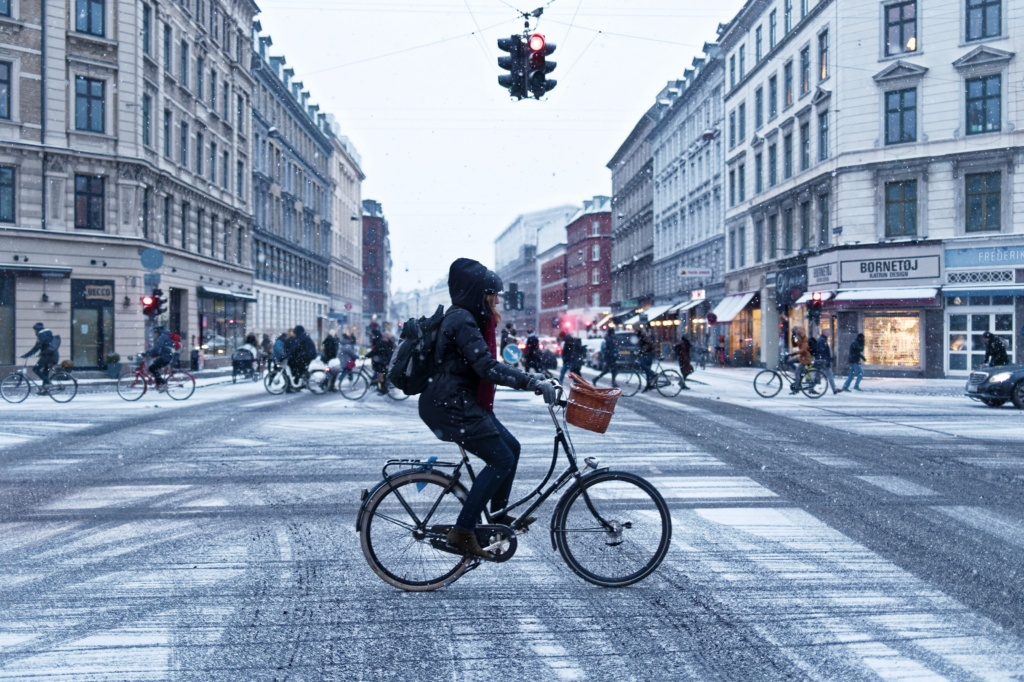## 目录

• 关于数据的更多信息-无聊的部分

• 柱形图

• 交互式地图

• 密度图

• 连接图

• 动画

• 结论

• 评论

## 关于数据的更多信息-无聊的部分

### 数据操作

```# Load journey data

f = 'journeys.csv'

date = j['date'].values
month = j['month'].values
year = j['year'].values
hour = j['hour'].values
minute = j['minute'].values
station_start = j['id_start'].values
station_end = j['id_end'].values```

```# Compute IsWeekday

weekday = np.zeros(len(date))
weekday[:] = np.nan
cnt = 0

for _year, _month, _date, _hour, _minute in zip(year, month, date, hour, minute):
_dt = datetime.datetime(_year, _month, _date, _hour, _minute)
_weekday = _dt.weekday()
weekday[cnt] = _weekday
cnt += 1

IsWeekday = weekday < 5
j['IsWeekday'] = IsWeekday

# Compute TimeSlice

j['TimeSlice'] = (hour*3 + np.floor(minute/20)).astype(int)```

```# Load station data

f = 'stations.csv'
station_id = stations['station_id'].values

# Extract valid journeys

valid = np.zeros(len(date))
valid[:] = False
cnt = 0

for _start, _end in zip(station_start, station_end):
if np.logical_and((_start in station_id), (_end in station_id)):
valid[cnt] = True
cnt += 1

j['Valid'] = valid```

```df = j[j["IsWeekday"] == True].drop(columns="IsWeekday")
df = df[df["Valid"] == True].drop(columns="Valid")
print('Ratio of valid journeys= {:.2f}%'.format(df.shape / j.shape * 100))```

### 柱形图

```grp_by_timeslice = df.groupby('TimeSlice').count().values[:,0]
plt.bar(range(0,72), grp_by_timeslice)
plt.xlabel('Time Slice')
plt.ylabel('Departures')
plt.show()```

[caption id="attachment_50081" align="aligncenter" width="1146"]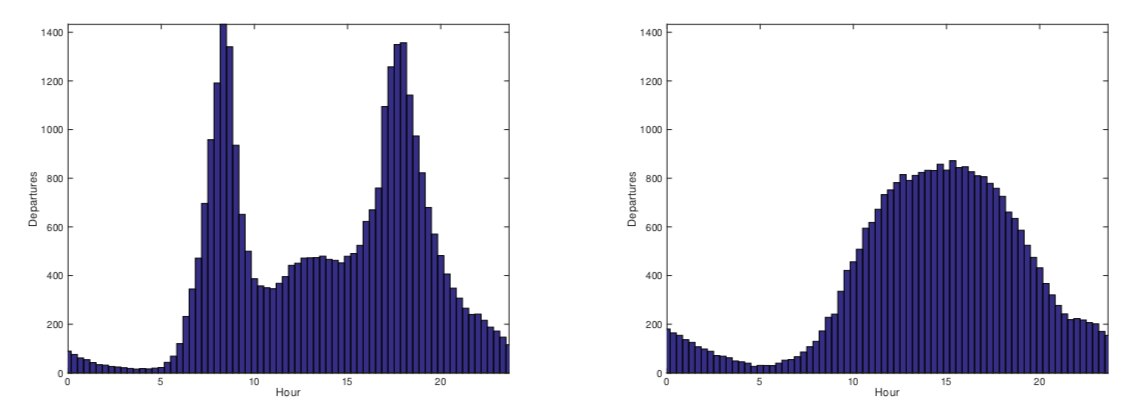平日（左）和周末（右）平均离港率[/caption]

[caption id="attachment_50082" align="aligncenter" width="1146"]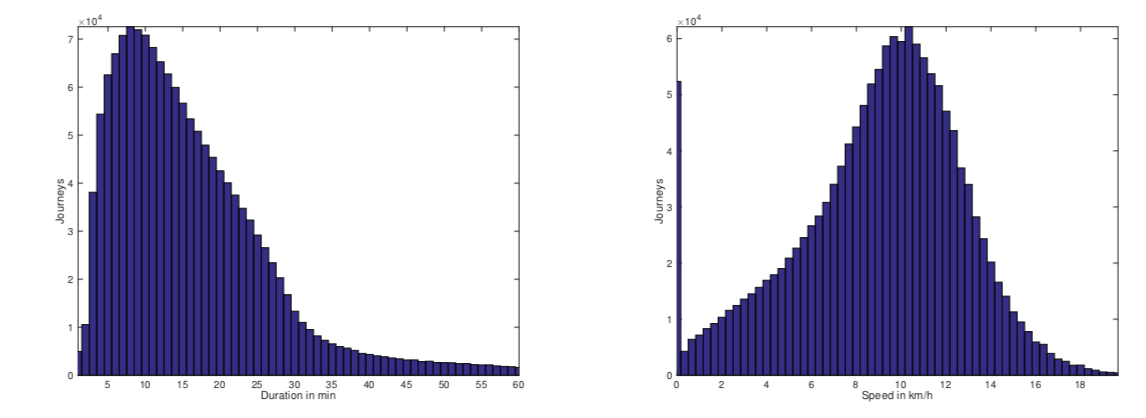行车时间分布(左)及速度分布(右)[/caption]

## 交互式地图

`\$ pip install folium==0.7.0`

```import folium

# Change colours

def color_change(c):
if(c < 15):
return('red')
elif(15 <= c < 30):
return('orange')
else:
return('green')
# Create base map

London = [51.506949, -0.122876]
map = folium.Map(location = London,
zoom_start = 12,
tiles = "CartoDB positron")

# Plot markers

for _lat, _lon, _cap, _name in zip(lat, lon, cap, name):
folium.CircleMarker(location = [_lat, _lon],
popup = "("+str(_cap)+") "+_name,
fill_color = color_change(_cap),
color = "gray",

f = 'map_station_cluster.html'
map.save(f)```

[caption id="attachment_50083" align="aligncenter" width="1660"]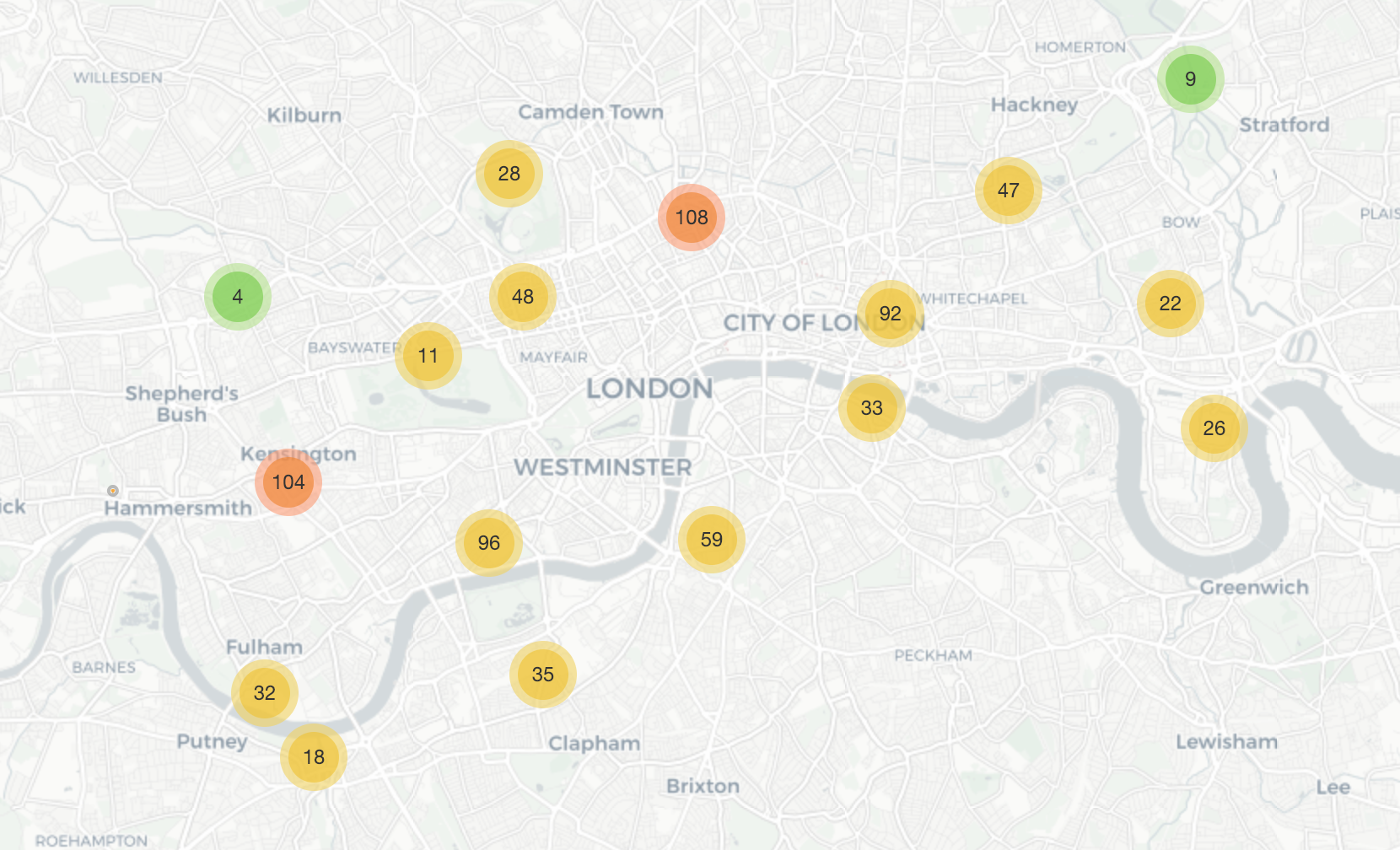站群图[/caption]放大时，它会自动取消聚集/展开：

[caption id="attachment_50084" align="aligncenter" width="1660"]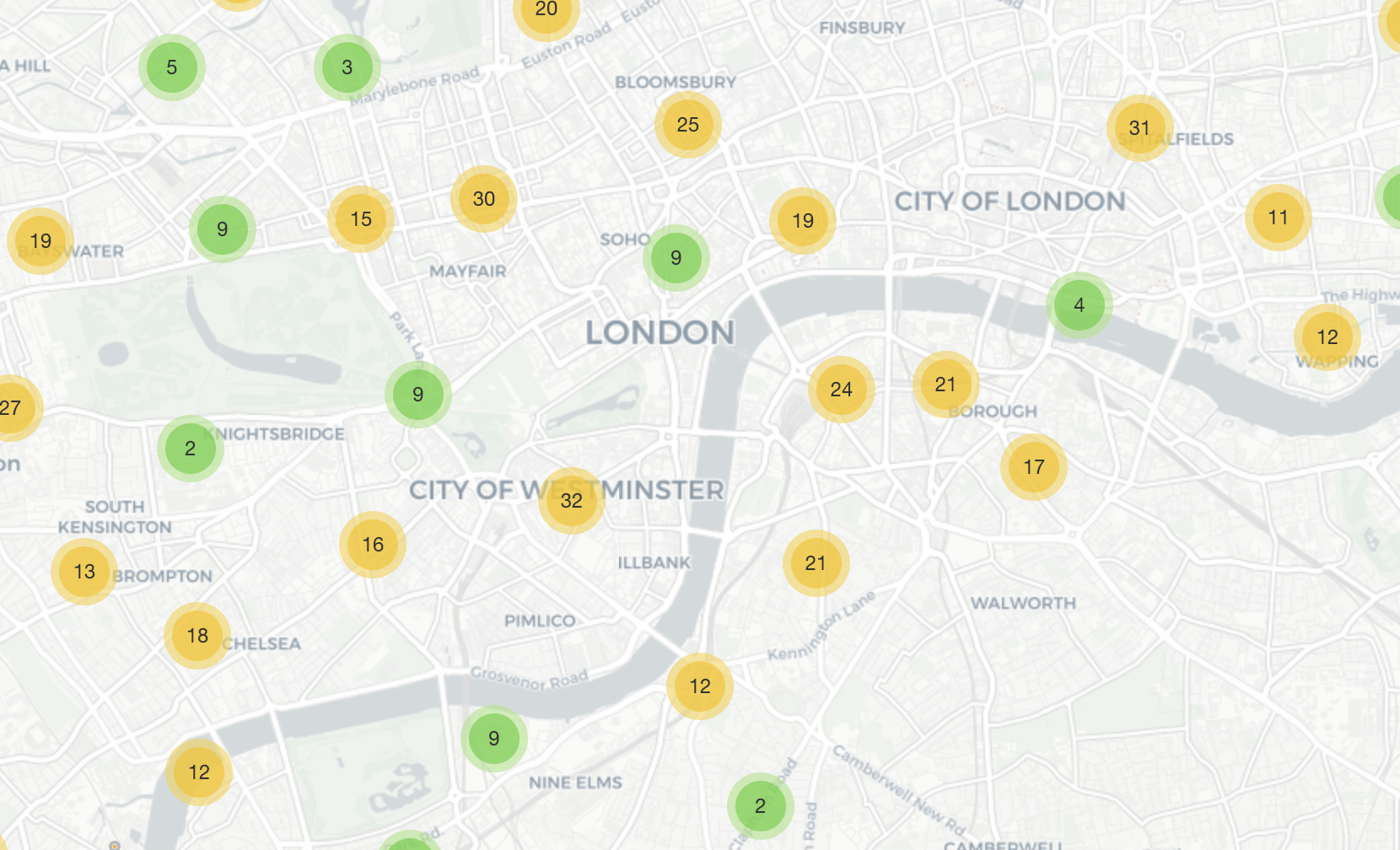站群地图-放大[/caption]

[caption id="attachment_50085" align="aligncenter" width="1660"]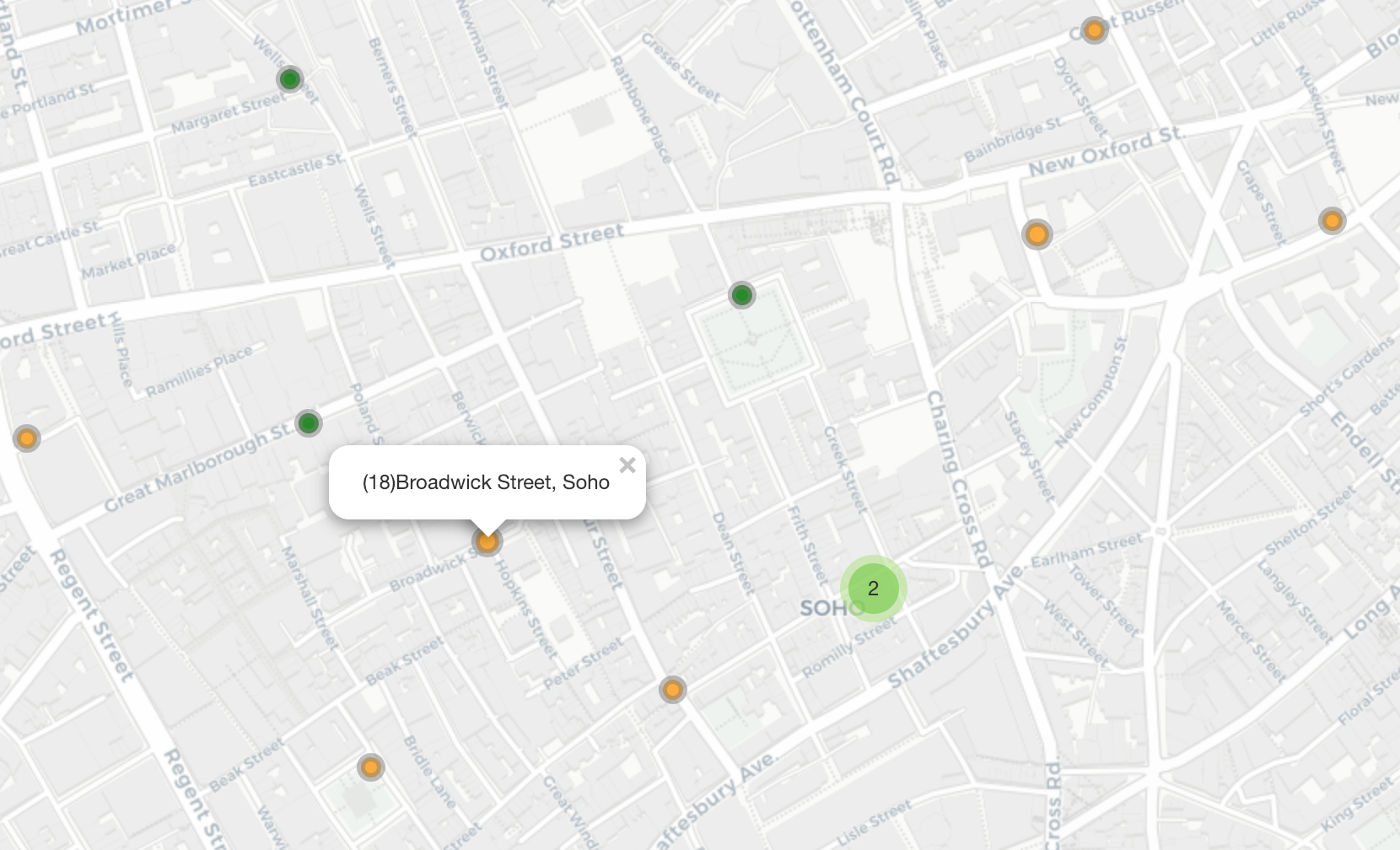站群图中的交互作用[/caption]AI热点
AI工具

Maluuba
20000~40000/月
Cisco
25000~30000/月 深圳市
PilotAILabs
30000~60000/年 深圳市